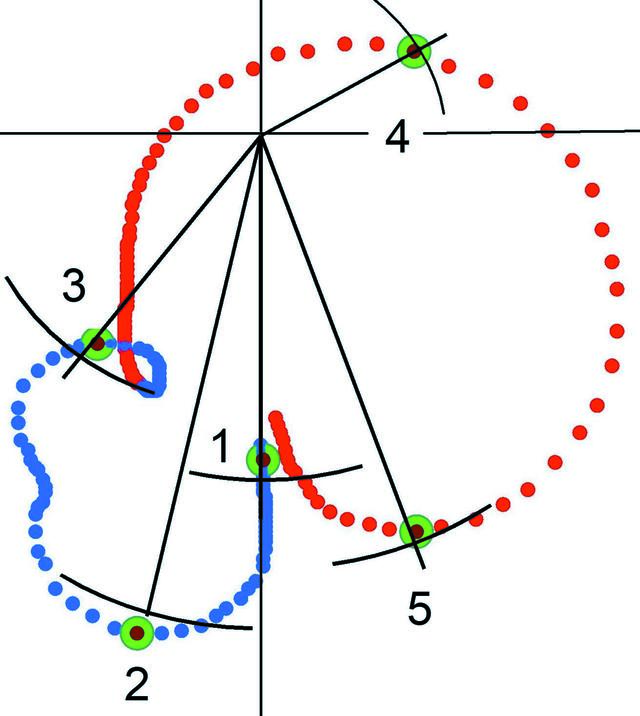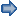disable zoom     view article Figure 5 Argand diagram of the 1,0,−1 reflection of trypsin. The root of the intensity measured at five wavelengths near the K-edge of sulfur is presented by radii intersected by a short section of a circle. The anomalous dispersion of X-ray scattering calculated from the model is shown by dots, which are blue near the edge of sulfate, and red near the edge of S atoms of trypsine. More widely spaced points correspond to strong anomalous dispersion. The calculated values to be compared with the experimental ones are the large green spheres. E1 = 2462 eV (5.035 Å), E2 = 2471 eV (5.017 Å), E3 = 2474 eV (5.012 Å), E4 = 2482 eV (4.995 Å), E5 = 2485 eV (4.989 Å) (Stuhrmann et al., 1997).FOUNDATIONSADVANCES
ISSN: 2053-2733
Volume 64| Part 1| December 2008| Pages 181-191